﻿ 基于Landsat OLI数据的青岛市土地利用分类和植被覆盖度反演 Land Use Classification and Vegetation Coverage Inversion Based on Landsat OLI Data in Qingdao

Geomatics Science and Technology
Vol. 07  No. 03 ( 2019 ), Article ID: 31389 , 7 pages
10.12677/GST.2019.73019

Land Use Classification and Vegetation Coverage Inversion Based on Landsat OLI Data in Qingdao

Yanzhen Liu, Yang Liu, Jia Guo

Shandong University of Science and Technology, Qingdao Shandong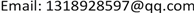Received: Jul. 4th, 2019; accepted: Jul. 17th, 2019; published: Jul. 24th, 2019ABSTRACT

Hierarchical classification method is a method to obtain classification rules by mining spatial data information, and vegetation coverage is an important parameter to characterize surface vegetation coverage. In this paper, based on the 2017 Landsat OLI image data of Qingdao city, land cover in the study area was classified and studied by stratified classification method. First of all, by making the necessary data preprocessing, the spectral characteristics of the image in the study area are analyzed and the image of NDVI, NDWI, NDBI index are calculated, and by using the stratified classification method we classify the land use in the research area. According to the results of land use classification and vegetation coverage inversion in the study area, it is advantageous to the planning of land utilization in Qingdao form, and it is of great importance to protect and improve the ecological environment of Qingdao and to plan the direction of urban development scientifically and reasonably.

Keywords:Landsat OLI Data, Land Use Classification, Hierarchical Classification, Vegetation Coverage1. 引言

2. 实验方法

2.1. 基于决策树的分层分类方法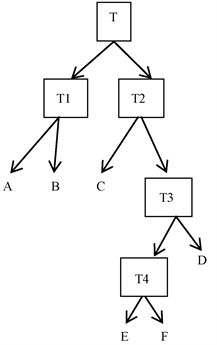Figure 1. Basic principles of decision tree classification algorithm

2.2. 精度评价

$K=\left({p}_{1}-{p}_{2}\right)/\left(1-{p}_{2}\right)$ (1)

${p}_{2}=\left({a}_{1}\ast {b}_{1}+{a}_{2}\ast {b}_{2}+\cdots +{a}_{0}\ast {b}_{0}\right)/{n}^{2}$ (2)

2.3. 植被覆盖度反演方法

${f}_{c}=\left(S-{S}_{\text{soil}}\right)/\left({S}_{\text{veg}}-{S}_{\text{soil}}\right)$ (3)

${f}_{c}=\left(\text{NDVI}-{\text{NDVI}}_{\text{soil}}\right)/\left({\text{NDVI}}_{\text{veg}}-{\text{NDVI}}_{\text{soil}}\right)$ (4)

3. 实验过程

3.1. 分类过程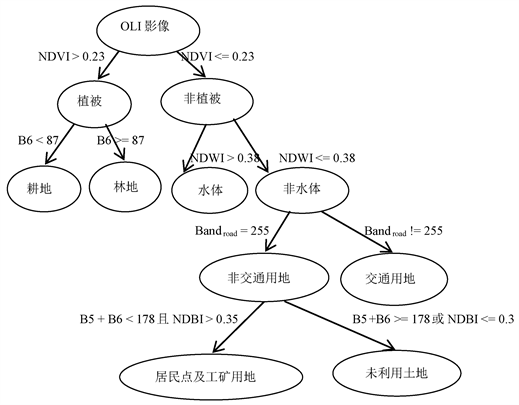Figure 2. Hierarchical classification map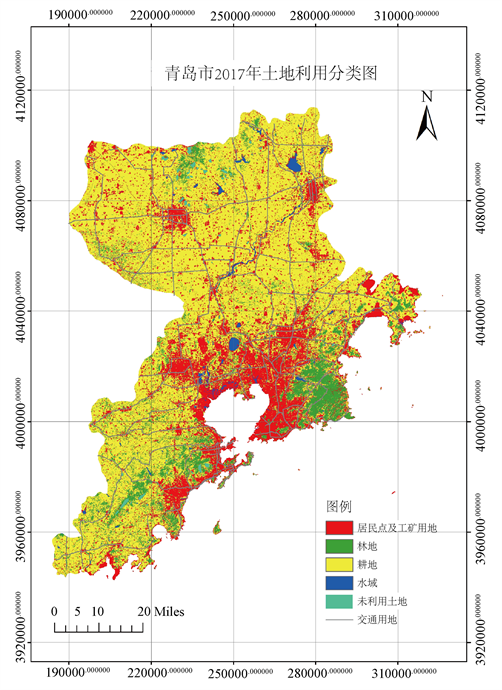Figure 3. Land use classification results map

3.2. 精度评价

$K=\frac{N{\sum }_{i=1}^{r}\text{\hspace{0.17em}}{x}_{ii}-{\sum }_{i=1}^{r}\left({x}_{i+}{x}_{+i}\right)}{{N}^{2}-{\sum }_{i=1}^{r}\left({x}_{i+}{x}_{+i}\right)}$ (5)

3.3. 植被覆盖度反演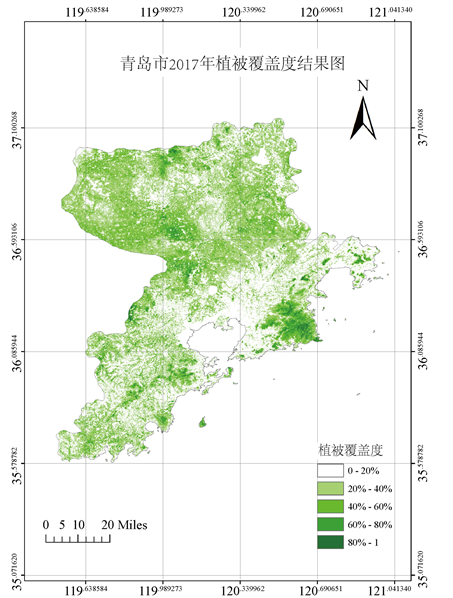Figure 4. Vegetation coverage inversion results map

4. 结束语

Land Use Classification and Vegetation Coverage Inversion Based on Landsat OLI Data in Qingdao[J]. 测绘科学技术, 2019, 07(03): 132-138. https://doi.org/10.12677/GST.2019.73019

1. 1. 张丽苏, 吴嘉平. 分层分区分景相结合的区域土地利用/覆盖分类方法——以浙江钱塘江流域分类为例[J]. 国土资源遥感, 2007, 19(3): 74-77.

2. 2. 凌涛, 李鹏, 左小清. 青岛市土地利用演化驱动力因素分析[J]. 安徽农业科学, 2016, 44(13): 257-262.

3. 3. Reyes, A., Solla, M. and Lorenzo, H. (2017) Comparison of Different Object-Based Clas-sifications in LandsatTM Images for the Analysis of Heterogeneous Landscapes. Measurement, 97, 29-37.

4. 4. Sinha, S., Sharma, L.K. and Nathawat, M.S. (2015) Improved Land-Use/Land-Cover Classification of Semi-Arid Deciduous Forest Landscape Using Thermal Remote Sensing. The Egyptian Journal of Remote Sensing and Space Science, 18, 217-233. https://doi.org/10.1016/j.ejrs.2015.09.005

5. 5. 明艳芳. 青岛市土地利用信息遥感提取方法[C]//智能信息技术应用学会. Proceedings of 2011 International Conference on Ecological Protection of Lakes-Wetlands-Watershed and Application of 3S Technology (EPLWW3S 2011 V3). 2011: 3.

6. 6. 李晓明, 常庆瑞, 张仁慧. 农牧交错地带土地利用分层分类方法研究——以靖边县为例[J]. 干旱地区农业研究, 2010, 28(5): 237-240.

7. 7. 李彤, 吴骅. 采用决策树分类技术对北京市土地覆盖现状进行研究[J]. 遥感技术与应用, 2004, 19(6): 485-487.

8. 8. 魏信. 基于决策树分类技术的北京城区土地利用分类研究[C]//2010 International Conference on Remote Sensing (ICRS 2010). Proceedings of 2010 International Conference on Remote Sensing (ICRS 2010) Volume 4. 2010: 4.

9. 9. 秦臻, 汪云甲, 王行风, 等. 基于ENVI的决策树方法在土地利用分类中的应用[J]. 金属矿山, 2011(2): 133-135+140.

10. 10. 王光远. 基于决策树的分类方法在土地利用分类中的应用[J]. 中国科技信息, 2013(3): 46-47.

11. 11. 郭力娜, 张梦华, 张永彬, 等. 唐山市区土地利用的Landsat 8影像分层分类[J]. 测绘科学, 2017, 42(10): 88-94+125.

12. 12. 李苗苗, 吴炳方, 颜长珍, 等. 密云水库上游植被覆盖度的遥感估算[J]. 资源科学, 2004, 26(4): 153-159.